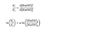# Chemical kinetics lab question

sergey_le
TL;DR Summary
tring to understand what went wrong when i did an experiment
last week i did a chemistry lab. i mixed NaOH 0.03M with equal part of methyl violet and mesured aborbance in a spectrophotometer. then i did the same thing with a 0.05M and methyl violet
i did today the graphs and got the k' =-0.1647 for the first solution and k'=-0.4022 for the second solution.( k'= k * OH conentration )
i was told to use the equation in the attached file. from what i understood the equation gives the reaction order and the instructor said we should get a=1.so i should get from this that only one reactent effects this reaction?
when i plug in my numbers i get a=1.74.
can anyone explain me the meaning of the equation , and what my result means? i guess that i didnt delute my NaOH wuth enough water and did the second expiement with 0.07M consentration instead of 0.05M (since if i put it in the equation instead of 0.05 iget a=0)

#### Attachments

•פורום.png
5 KB · Views: 160
Last edited by a moderator: